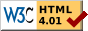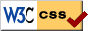Search LinuxSupport
Large Disk HOWTO: Details## 10.1 IDE details - the seven geometries

The IDE driver has five sources of information about the geometry. The first (G_user) is the one specified by the user on the command line. The second (G_bios) is the BIOS Fixed Disk Parameter Table (for first and second disk only) that is read on system startup, before the switch to 32-bit mode. The third (G_phys) and fourth (G_log) are returned by the IDE controller as a response to the IDENTIFY command - they are the `physical' and `current logical' geometries.

On the other hand, the driver needs two values for the geometry: on the one hand G_fdisk, returned by a `HDIO_GETGEO` ioctl, and on the other hand G_used, which is actually used for doing I/O. Both G_fdisk and G_used are initialized to G_user if given, to G_bios when this information is present according to CMOS, and to to G_phys otherwise. If G_log looks reasonable then G_used is set to that. Otherwise, if G_used is unreasonable and G_phys looks reasonable then G_used is set to G_phys. Here `reasonable' means that the number of heads is in the range 1-16.

To say this in other words: the command line overrides the BIOS, and will determine what `fdisk` sees, but if it specifies a translated geometry (with more than 16 heads), then for kernel I/O it will be overridden by output of the IDENTIFY command.

Note that G_bios is rather unreliable: for systems booting from SCSI the first and second disk may well be SCSI disks, and the geometry that the BIOS reported for sda is used by the kernel for hda. Moreover, disks that are not mentioned in the BIOS Setup are not seen by the BIOS. This means that, e.g., in an IDE-only system where hdb is not given in the Setup, the geometries reported by the BIOS for the first and second disk will apply to hda and hdc.

## 10.2 SCSI details

The situation for SCSI is slightly different, as the SCSI commands already use logical block numbers, so a `geometry' is entirely irrelevant for actual I/O. However, the format of the partition table is still the same, so `fdisk` has to invent some geometry, and also uses `HDIO_GETGEO` here - indeed, `fdisk` does not distinguish between IDE and SCSI disks. As one can see from the detailed description below, the various drivers each invent a somewhat different geometry. Indeed, one big mess.

If you are not using DOS or so, then avoid all extended translation settings, and just use 64 heads, 32 sectors per track (for a nice, convenient 1 MiB per cylinder), if possible, so that no problems arise when you move the disk from one controller to another. Some SCSI disk drivers (aha152x, pas16, ppa, qlogicfas, qlogicisp) are so nervous about DOS compatibility that they will not allow a Linux-only system to use more than about 8 GiB. This is a bug.

What is the real geometry? The easiest answer is that there is no such thing. And if there were, you wouldn't want to know, and certainly NEVER, EVER tell `fdisk` or LILO or the kernel about it. It is strictly a business between the SCSI controller and the disk. Let me repeat that: only silly people tell `fdisk`/LILO/kernel about the true SCSI disk geometry.

But if you are curious and insist, you might ask the disk itself. There is the important command READ CAPACITY that will give the total size of the disk, and there is the MODE SENSE command, that in the Rigid Disk Drive Geometry Page (page 04) gives the number of cylinders and heads (this is information that cannot be changed), and in the Format Page (page 03) gives the number of bytes per sector, and sectors per track. This latter number is typically dependent upon the notch, and the number of sectors per track varies - the outer tracks have more sectors than the inner tracks. The Linux program `scsiinfo` will give this information. There are many details and complications, and it is clear that nobody (probably not even the operating system) wants to use this information. Moreover, as long as we are only concerned about `fdisk` and LILO, one typically gets answers like C/H/S=4476/27/171 - values that cannot be used by `fdisk` because the partition table reserves only 10 resp. 8 resp. 6 bits for C/H/S.

Then where does the kernel `HDIO_GETGEO` get its information from? Well, either from the SCSI controller, or by making an educated guess. Some drivers seem to think that we want to know `reality', but of course we only want to know what the DOS or OS/2 FDISK (or Adaptec AFDISK, etc) will use.

Note that Linux `fdisk` needs the numbers H and S of heads and sectors per track to convert LBA sector numbers into c/h/s addresses, but the number C of cylinders does not play a role in this conversion. Some drivers use (C,H,S) = (1023,255,63) to signal that the drive capacity is at least 1023`*`255`*`63 sectors. This is unfortunate, since it does not reveal the actual size, and will limit the users of most `fdisk` versions to about 8 GiB of their disks - a real limitation in these days.

In the description below, M denotes the total disk capacity, and C, H, S the number of cylinders, heads and sectors per track. It suffices to give H, S if we regard C as defined by M / (H`*`S).

By default, H=64, S=32.

aha1740, dtc, g_NCR5380, t128, wd7000:

H=64, S=32.

aha152x, pas16, ppa, qlogicfas, qlogicisp:

H=64, S=32 unless C > 1024, in which case H=255, S=63, C = min(1023, M/(H`*`S)). (Thus C is truncated, and H`*`S`*`C is not an approximation to the disk capacity M. This will confuse most versions of `fdisk`.) The `ppa.c` code uses M+1 instead of M and says that due to a bug in `sd.c` M is off by 1.

H=64, S=32 unless C > 1024 and moreover the `> 1 GB' option in the BIOS is enabled, in which case H=255, S=63.

aha1542:

Ask the controller which of two possible translation schemes is in use, and use either H=255, S=63 or H=64, S=32. In the former case there is a boot message "aha1542.c: Using extended bios translation".

aic7xxx:

H=64, S=32 unless C > 1024, and moreover either the "extended" boot parameter was given, or the `extended' bit was set in the SEEPROM or BIOS, in which case H=255, S=63. In Linux 2.0.36 this extended translation would always be set in case no SEEPROM was found, but in Linux 2.2.6 if no SEEPROM is found extended translation is set only when the user asked for it using this boot parameter (while when a SEEPROM is found, the boot parameter is ignored). This means that a setup that works under 2.0.36 may fail to boot with 2.2.6 (and require the `linear' keyword for LILO, or the `aic7xxx=extended' kernel boot parameter).

buslogic:

H=64, S=32 unless C >= 1024, and moreover extended translation was enabled on the controller, in which case if M < 2^22 then H=128, S=32; otherwise H=255, S=63. However, after making this choice for (C,H,S), the partition table is read, and if for one of the three possibilities (H,S) = (64,32), (128,32), (255,63) the value endH=H-1 is seen somewhere then that pair (H,S) is used, and a boot message is printed "Adopting Geometry from Partition Table".

fdomain:

Find the geometry information in the BIOS Drive Parameter Table, or read the partition table and use H=endH+1, S=endS for the first partition, provided it is nonempty, or use H=64, S=32 for M < 2^21 (1 GiB), H=128, S=63 for M < 63`*`2^17 (3.9 GiB) and H=255, S=63 otherwise.

in2000:

Use the first of (H,S) = (64,32), (64,63), (128,63), (255,63) that will make C <= 1024. In the last case, truncate C at 1023.

seagate:

Read C,H,S from the disk. (Horrors!) If C or S is too large, then put S=17, H=2 and double H until C <= 1024. This means that H will be set to 0 if M > 128`*`1024`*`17 (1.1 GiB). This is a bug.

ultrastor and u14_34f:

One of three mappings ((H,S) = (16,63), (64,32), (64,63)) is used depending on the controller mapping mode.

If the driver does not specify the geometry, we fall back on an educated guess using the partition table, or using the total disk capacity.

Look at the partition table. Since by convention partitions end on a cylinder boundary, we can, given `end = (endC,endH,endS)` for any partition, just put H = `endH+1` and S = `endS`. (Recall that sectors are counted from 1.) More precisely, the following is done. If there is a nonempty partition, pick the partition with the largest `beginC`. For that partition, look at `end+1`, computed both by adding `start` and `length` and by assuming that this partition ends on a cylinder boundary. If both values agree, or if `endC` = 1023 and `start+length` is an integral multiple of `(endH+1)*endS`, then assume that this partition really was aligned on a cylinder boundary, and put H = `endH+1` and S = `endS`. If this fails, either because there are no partitions, or because they have strange sizes, then look only at the disk capacity M. Algorithm: put H = M/(62`*`1024) (rounded up), S = M/(1024`*`H) (rounded up), C = M/(H`*`S) (rounded down). This has the effect of producing a (C,H,S) with C at most 1024 and S at most 62.LinuxSupport.org.uk was designed and built by Paul Vigay. In Pauls absence this site has now been taken over and updated by Bob Ruskin.Latest Updates | Copyright | Disclaimer | Privacy policy